# Wind Velocity Pressure Calculation for Wind Load Analysis

### Velocity Pressure for Wind Load

Velocity pressure, qz or qh, shall be calculated from the formula: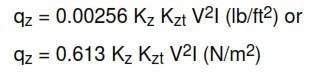where

I = Importance factor as per Table III (ASCE 7-95 Table 6-2)
Kz = Velocity pressure exposure coefficient as per Table V (ASCE 7-95 Table 6-3).
*Kzt  = Topographic factor.

*Wind speed-up over isolated hills and escarpments (that constitutes abrupt changes in the general  topography) shall be considered for buildings and other structures sited on the upper half of hills and ridges or near the edge of escarpments by the topographic factor Kzt. This shall apply to isolated hills or
escarpments located in exposure B, C, and D where the upward terrain is free of topographic features for a distance equal to 50 H or 1 mile (1.6 km), whichever is smaller, as measured from the point at which H is determined.

## Wind Velocity Pressure Calculation for Wind Load Analysis

For the appropriate topographic conditions, the determination of Kzt shall be in accordance with note below and Figure A1 (ASCE 7-95, Figure 6-2).

Note (Copied from ASCE 7-95, 6.5.5): The provisions of this section shall apply to isolated hills or escarpments located in exposure B, C, or D where the upwind terrain is free of such topographic features for a distance equal to 50H or 1 mile, whichever is smaller, as measured from the point at which H is
determined. Wind speed-up over isolated hills and escarpments that constitute abrupt changes in the general topography shall be considered for buildings and other structures sited on the upper half of hills and ridges or near the edges of escarpments, illustrated in Figure A1 (ASCE 7-95, Figure 6-2) by using
factor Kzt.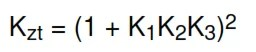where K1, K2 and K3 are given in Figure A1 (ASCE Figure 6-2). The effect of wind speed-up shall not be required to be considered when H/Lh < 0.2, or when H < 15 ft (4.5 m) for exposure D, or < 30 ft (9 m) for exposure C, or < 60 ft (18 m) for all other exposures.

Table V (ASCE 7-95 Table 6-3) – Velocity Pressure Exposure Coefficients, Kh  and Kz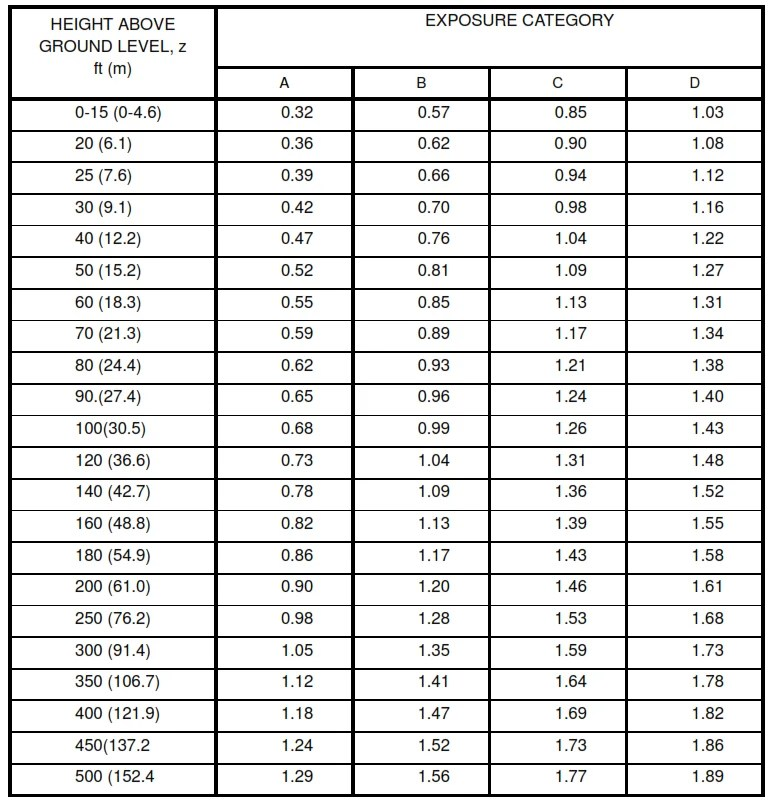Table V (ASCE 7-95 Table 6-3) – Velocity Pressure Exposure Coefficients, Kh  and Kz

Notes:

1. Linear interpretation for intermediate values of height z is acceptable.
2. For values of height z greater than 500 ft (152.4m), Kz shall be calculated from Eq.(C3) of ASCE-7-95 or as modified by the latest revision of ASCE-7.
3. Exposure categories C and D are defined in section 4.4.1 and 4.4.2. For exposure categories A and B, refer to section 6.5.3 of ASCE-7-95 (or as modified in latest revision).
4. Values for qh or qz , in lb/ft2 , are listed in table VI (exposure C) and table VII (exposure D) for various wind velocities (V), and based on Kzt = 1.0 & I = 1.00.

To obtain the values of qh or qz , corresponding to exposure C (or D), select wind velocity, V from Table I for respective site location, enter this V value in table VI (or VII for exposure D) and select qz or qh at the the desired height, z or h. Values from tables VI and VII can be linearly scaled and multiplied by the applicable values of I  and Kzt to get the actual wind pressure in lb/ft2.

5. Table Va gives velocity pressure coefficients Kh and Kz corresponding to building heights in meters. Tables VIa (exposure C) and VIIa (exposure D) gives wind pressure qz for plants based on Kzt =1.0, I = 1.15. The actual wind pressure qz and qh shall be obtained for plants by multiplying the interpolated pressure value in the tables with the actual calculated Topographical pressure coefficient value, Kzt = (1+K1k2k3)².

Velocity Pressure Exposure Coefficient Kh Table Va and Kz (interpolated for height z or h in metric unit)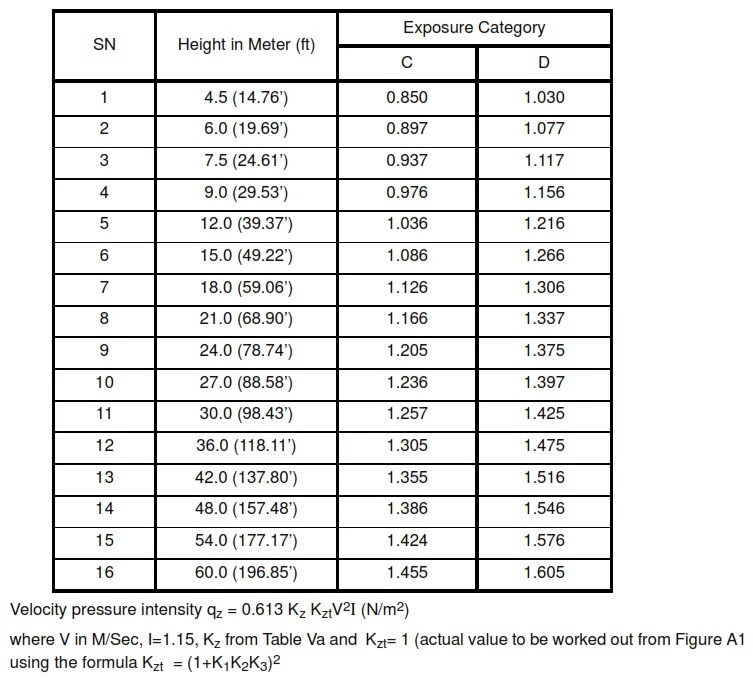Velocity Pressure Exposure Coefficient Kh Table Va and Kz (interpolated for height z or h in metric unit)

Table VI –  Velocity Pressures, qz (or qh for Z = h) lb/ft2 (exposure C)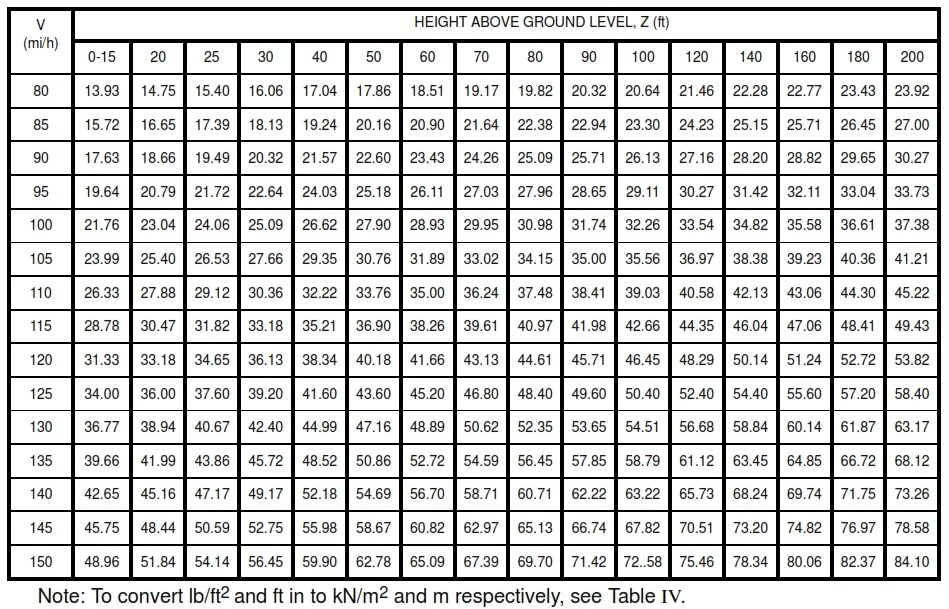Table VI –  Velocity Pressures, qz (or qh for Z = h) lb/ft2 (exposure C)

Table VIa – Showing Velocity Pressure Intensity qz or qh for exposure CTable VIa – Showing Velocity Pressure Intensity qz or qh for exposure C

Table VII – Velocity Pressures, qz (or qh for Z = h) lb/ft2 (exposure D)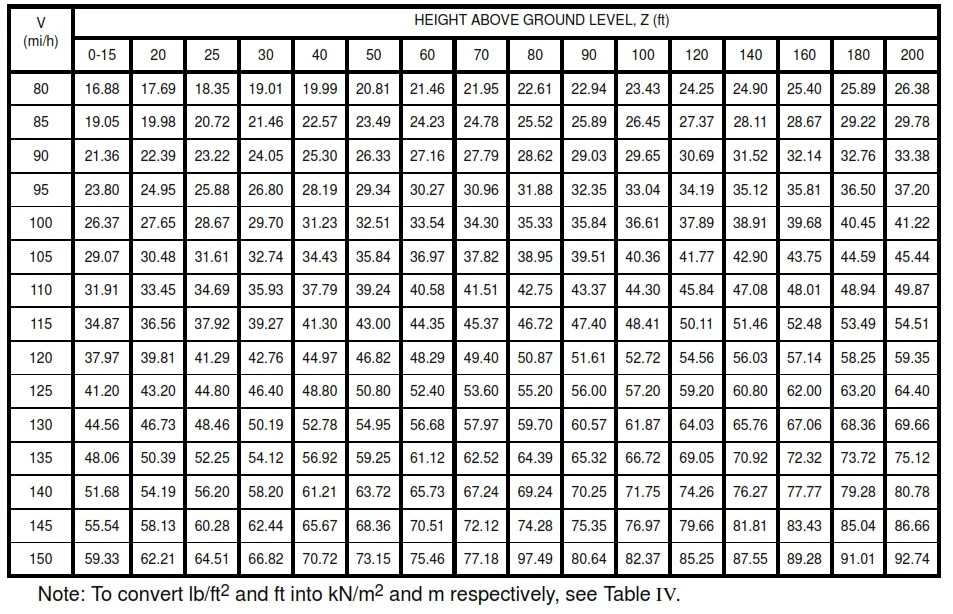Table VII – Velocity Pressures, qz (or qh for Z = h) lb/ft2 (exposure D)

Table VII a Showing Velocity Pressure Intensity qz or qh for exposure DTable VII a Showing Velocity Pressure Intensity qz or qh for exposure D

## Gust Effect Factors

For main wind-force resisting systems of buildings and other structures, and for components and cladding of open buildings and other structures, the value of the gust effect factor, G, shall be 0.85 for exposures C and D.

paktechpoint.com

#### You may also like...

error: tected !!Content is pro